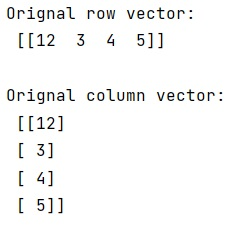# Differentiating between row and column vectors

Learn, how to differentiate between row and column vectors in Python?
Submitted by Pranit Sharma, on January 13, 2023

NumPy is an abbreviated form of Numerical Python. It is used for different types of scientific operations in python. Numpy is a vast library in python which is used for almost every kind of scientific or mathematical operation. It is itself an array which is a collection of various methods and functions for processing the arrays.

## Vectors - row vs column

Many times, we create a 1-dimensional vector and observe that the original vector and its transpose are equal.

A one-dimensional sequence of numbers is neither a row nor a column vector. A row or column vector is a two-dimensional array (in which one of the two dimensions is 1).

Hence, we can make the distinction explicit by adding another dimension to the array. For this purpose, we will first create an actual row or column vector. Then we will reshape the current vector and finally, we can explicitly create a row or column by making it transpose.

Let us understand with the help of an example,

## Python code to demonstrate the example for differentiating between row and column vectors

```# Import numpy
import numpy as np

# Creating a row vector
row = np.array([12, 3, 4, 5], ndmin=2)

# Display original row vector
print("Orignal row vector:\n",row,"\n")

# Creating a column vector
col = row.T

# Display column vector
print("Orignal column vector:\n",col,"\n")
```

Output:What's New (MCQs)

Top Interview Coding Problems/Challenges!

IncludeHelp's Blogs

Languages: » C » C++ » C++ STL » Java » Data Structure » C#.Net » Android » Kotlin » SQL
Web Technologies: » PHP » Python » JavaScript » CSS » Ajax » Node.js » Web programming/HTML
Solved programs: » C » C++ » DS » Java » C#
Aptitude que. & ans.: » C » C++ » Java » DBMS
Interview que. & ans.: » C » Embedded C » Java » SEO » HR
CS Subjects: » CS Basics » O.S. » Networks » DBMS » Embedded Systems » Cloud Computing
» Machine learning » CS Organizations » Linux » DOS
More: » Articles » Puzzles » News/Updates